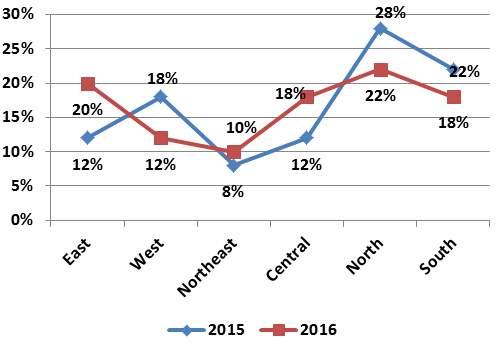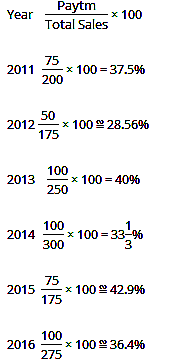# High Level Data Interpretation Practice Questions

High Level Data Interpretation Practice Questions
Directions (1 – 5): Study the following table and chart carefully and answer the questions given beside:
The following table shows the sales figures of Patanjali (in Rs. million) for the period of 2012 to 2017
 Months 2012-13 2013-14 2014-15 2015-16 2016-17 April 7.2 10.4 14.6 24.4 41.10 May 9.2 11.7 18.3 26.5 47.84 June 12.7 20.6 20.4 32.2 47.96 July 15.6 14.6 22.4 33.8 102.96 August 10.5 15.4 21.3 41.03 53.3 September 8.8 16.2 18.7 55.06 57.2 October 14.8 13.8 20.4 36.2 63.2 November 20.6 12.5 26.6 47.08 71.05 December 24.4 18.2 22.5 62.8 45.3 January 8.4 19.4 27.8 45.07 13.02 February 7.8 14.8 23.04 48.9 49.92 March 9.7 12.4 26.06 47.3 46.4
The following pie chart shows the contribution of different products to the sales figure 2016-17
1. For which month in 2016-17 was the annual growth rate for sales figures the highest with respect to corresponding month of 2012-13?
a) September
b) May
c) February
d) November
e) July
2. If the profit margins, as percentage of sales, on Cow Ghee, Soap, Dant kanti and Kesh Kanti in 2016-17 are 23%, 16%, 25% and 20% respectively, which of these products contributed the maximum to the profit for the year 2016-17?
a) Dant Kanti
b) Kesh Kanti
c) Cow Ghee
d) Soap
e) Can’t be determined
3. In which year was the growth rate sale in a month over that of the previous month the highest?
a) 2012 – 13
b) 2013 – 14
c) 2014 – 15
d) 2015 – 16
e) 2016 – 17
4. If the profit margins, as percentage of sales, on Cow Ghee, Soap, Dant kanti and Kesh Kanti in 2013-14 are 18%, 12%, 22% and 16% respectively and sales from these products form 32%, 25%, 20% and 15% respectively, what is the approximate profit contributed by these products (in Rs. million)?
a) 35
b) 28
c) 30
d) 25
e) 31
5. What would be the sale in January 2017-18, if we take the double of the percentage increase in the sale happened in 2016-17 in comparison to January 2012-13?
a) Rs.27.34 m
b) Rs.22.87 m
c) Rs.28.34 m
d) Rs.21.52 m
e) Rs.23.84 m
Directions (6 – 10): Study the following information carefully to answer the question given beside:
The yearly sales turnover of e-commerce companies Flipkart, Shopclues, Paytm and Snapdeal in crores from 2011 to 2016.6. In which of the following years is Paytm's profit the highest, if it makes 25% profit on its sales turnover in each year?
a) 2015
b) 2011 and 2015
c) 2012, 2015 and 2016
d) 2013, 2014 and 2016
e) 2014 and 2016
7. In which of the given years is the sales turnover of Flipkart as a percentage of the combined sales turnover of Shopclues and Snapdeal the least?
a) 2016
b) 2013
c) 2011
d) 2015
e) 2012
8. In how many years was the sales turnover of Paytm more than one-third of the total sales turnover of the four companies?
a) 1 year
b) 3 years
c) 5 years
d) 6 years
e) None of these
9. In how many years, from 2012 to 2016, did the sales turnover of Flipkart as a percentage of the total sales turnover of the four companies increases over that of its immediate preceding year?
a) 1
b) 2
c) 3
d) 4
e) None
15. Which of the following statements must be true?
a) The sales of Snapdeal, as a percentage of total sales, were the same for four years
b) The ratio of the total sales of Paytm to the total sales of Flipkart, from 2011 to 2016 was 19 : 20
c) The total sales of Shopclues from 2011 to 2016 were approximately 12.7% of the total sales of all the four companies for given period.
d) For each year, the sales of Shopclues were always more than the sales of Snapdeal.
e) None of the above is true
Directions (11 – 15): Study the following line graph carefully and answer the given questions.
The following line-graph shows the percentage of aspirants appearing in SSC examination from different zones in two successive years. The total number of aspirants appearing in 2015 and 2016 was 4.5 lakh and 4.65 lakh respectively.11. For which of the following zones is the difference between the number of aspirants appearing in 2015 and 2016 the maximum?
a) West zone
b) North zone
c) Central zone
d) East zone
e) None of these
12. If 15% of the number of aspirants qualify from Central zone in 2015 for the main exam, then the total number of qualified aspirants from Central zone is approximately what per cent of the total number of appeared aspirants in that year?
a) 2%
b) 3%
c) 4%
d) 1%
e) None of these
13. What is the difference between the total number of aspirants who appear from East zone in 2016 and the number of aspirants who appear from North zone in 2015 in the exam?
a) 18500
b) 19000
c) 19500
d) 20000
e) None of these
14. What is the average number of aspirants who appear from East, West and Central zones together in 2015 and North and South zone together in 2016?
a) 155500
b) 157500
c) 160000
d) 160500
e) None of these
15. What is the percentage increase in the number of aspirants in the exam from the Central zone in 2016 in comparison to the previous year from the same zero?
a) 55%
b) 70%
c) 66%
d) 50%
e) None of these
Solutions:
1. E) By just looking at the table we can observe that it is the month of July in which the highest growth sale happens.
Reqd Growth % = (102.96 – 15.6)/15.6 * 100 = 87.36/15.6 * 100 = 560
2. C) Let sales in 2016-17 be Rs. x
Profit contributed by Cow Ghee
27% of 23% of x = 0.0621x
Profit contributed by Soap
19% of 16% of x = 0.0304x
Profit contributed by Dant Kanti
22% of 25% of x = 0.055x
Profit conributed by Kesh Kanti
15% of 20% of x = 0.030x
Hence, Cow Ghee contributed the maximum.
3. E) A close observation of the table, we get that it is the month of February 2016-17 in which the growth rate was maximum, ie. approximate 283%.
As per the given information,
(49.92 – 13.02)/13.02 * 100 = 283.41%
4. B) Sales for 2013-14 = Rs. 180 million
Profit = [(0.32 × 0.18) + (0.25 × 0.12) + (0.20 × 0.22) + (0.15 × 0.16)] of 180
= [0.0576 + 0.03 + 0.044 + 0.024] of 180
= 0.1556 × 180 ≈ 28 million
5. A) Percentage increase in the month of January from 2012-13 to 2016-17 = (13.02 – 8.4)/8.4 * 100 = 55%
Now, the effective percentage increase rate, as per the question
55 × 2 = 110%
Therefore, the sale in Jan, 2017-18 will be = (100 + 110)% of 13.02 = 210% of 13.02 = Rs. 27.34 million
6. D) As the profit percentage in each year is the same, Paytm will have the maximum profit in that year which has the maximum sales turnover.
Year               Sales Turnover
2011             175 – 100 = ₹ 75 Crores
2012             150 – 100 = ₹ 50 Crores
2013             225 – 125 = ₹ 100 Crores
2014             250 – 150 = ₹ 100 Crores
2015             150 – 75 = ₹ 75 Crores
2016             200 – 100 = ₹ 100 Crores
Hence, option D is a correct choice.
7. A)Hence, the required percentage was the least in the year 2016.
8. E)Hence, it is during four years - 2011, 2013, 2015 & 2016 (% values greater than 33.33%) that the turnover of Paytm is more than one third of the total sales. (The year 2014 is not counted, as Paytm's sales was exactly one-third of the total sales, not more than one-third)
Hence, option E is correct.
9. A)An increase in sales over a preceding year took place only from 2011 to 2012 i.e. from 37.5% to 42.86%
Hence, option A is the correct answer.
10. C) Verifying the options one by one
From option A: During four years, the total sales of Snapdeal were the same, i.e. 25 crores, but as the total sales of all four companies was different in each year, hence option A is false.
From option B: For the given period,
Total sales of Paytm = 75 + 50 + 100 + 100 + 75 + 100 = 500.
Total sales of Flipkart = 75 + 75 + 100 + 100 + 50 + 75 = 475.
The required ratio is 500 : 475 = 20 : 19, not 19 : 20. Hence option B is false
From option C: Total sales of all the four companies for given period = 200 + 175 + 250 + 300 + 175 + 275 = 1375
Total sales of Shopclues for the given period = 25 × 5 + 50 = 175
Required % = 175/1375 * 100 = 12.72%
From option D: The sales of Shopclues were less than the sales of Snapdeal in 2016, Hence option D is false.
Hence, option C is correct.
11. E) From the graph, it is clear that in East zone the difference in % values is the maximum, ie 20% - 12% = 8%. So, the difference between the number of appearing students in 2015 and 2016 will be the maximum in East Zone.
12. A) Reqd % = {[450000 × (12/100) × (15/100)]/450000} × 100
= (9/500)×100 = 1.8%, So approx = 2%
13. E) Reqd Difference,
= 450000 × (28/100) 465000 × (20/100)
= 126000 93000 = 33000
14. E) Required Average
= [(12+18+12)% of 4.5 lakh + (22+18)% of 4.65 lakh]/2
= (42% of 4.5 lakh + 40% of 4.65 lakh)/2
= [(1.89+1.86)/2] lakh = 1.875 lakh = 187500
15. A) Required Percentage
= [(18% of 4.65 lakh – 12% of 4.5 lakh)/12% of 4.5 lakh] × 100
= (83.70 – 54)/54 × 100 = 29.7/54 × 100 = 55%